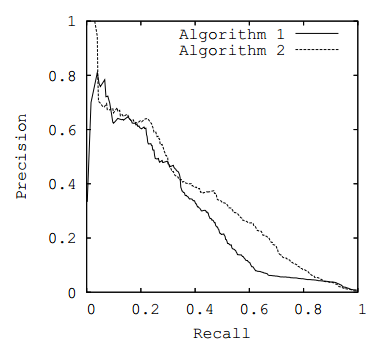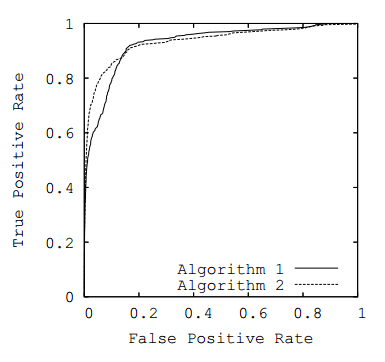PR-AUC 和 ROC-AUC 机器学习的重要评价指标，常用于二元决策问题。

## Define of PR and ROC

predicted positivepredicted negative
actual positiveTPFN
actual negativeFPTN

### PR

P 为 precision，预测中的正例与所有预测之间的比值，表示正例在预测中的精度；

R 为 recall，预测中的正例与数据集中所有正例之间的比值，表示正例的查全率；#### Nature of RP Curve

• 当预测总数小于 $A$ 时，由于预测总数较少，随着预测总数的增加，预测正例的增加 $TP_{rate}$ 大于一定的预测反例的增加 $FP_{rate}$，此时可能出现 P 增大，表现为增函数
• 当预测总数小于 $A$ 时，随着预测总数的增加，有可能因为算法性能差，预测正例的增加 $TP_{rate}$ 不大于一定的预测反例增加 $FP_{rate}$ ，导致 P 减少，表现为减函数
• 当预测总数大于 $A$ 后，随着预测总数的增加，预测正例数量 $TP$ 趋于稳定，预测反例 $FP$ 增多，导致 P 减小，表现为减函数

RP 曲线为预测正例准确度和预测正例查全率之间的映射关系，当预测总数足够大时，查全率可达到1，而此时预测反例很多，预测正例准确度趋于0，即曲线收敛于点 $(1,0)$

#### AUC

area under curve。当算法在较小的预测总数时能达到较高的查全率和正例准确度时，表示算法的性能好。此时 PR 曲线趋于右上方，即曲线的性能越好，则此时应该尽可能”右凸“，曲线下面积尽可能大。

### ROC

TPR 为 true positive rate，预测中的正例与数据集中所有正例之间的比值，可表示正例查全率；

FPR 为 false positive rate，预测中的反例与数据集中所有反例之间的比值，表示反例的查全率；#### Nature of ROC Curve

• 若预测的总数变多，而正例数不变，反例数变多，ROC 为水平，则要么是该算法已经达到较高的正例查全率（曲线位于右上方），要么表示该算法性能较差，无法提高正例查全率
• 若预测的总数变多，反例数不增多，而正例增多，表示预测总数还不足够多，未能达到算法的预测能力容量，此时曲线往往应该处于 x 较小的地方，且比较陡峭
• 随着预测总数量的变多，算法不应存在预测正例数减少或预测反列数减少的情况。

### Relationship Between PR and ROC

• 对于给定 positive 和 negative 样本的数据集，若 Recall 不等于 0，则 ROC 空间和 PR 空间上存在唯一对应的两条曲线，这两条曲线也唯一确定了一个 confusion matrices
• ROC 空间上某曲线完全大于等于另一条曲线当且仅当该曲线在 PR 空间的对应曲线也完全大于等于另一条曲线（a curve dominates in ROC space if and only if it dominates in PR space）
• 一个算法优化 ROC-AUC 不等于优化了 PR-AUC

## Example

method 1： 检索出 100 份文档，其中有 90 份匹配正确，其余匹配错误

method 2： 检索出 2000 份文档，其中有 90 份匹配正确，其余匹配错误

### PR and ROC for method 1

predicted positivepredicted negative
actual positive (100)TP = 90FN = 10
actual negative (999,900)FP = 10TN = 999,890

PR for method 1:

P = 90 / (90 + 10) = 0.9

R = 90 / (90 + 10) = 0.9

ROC for method 1:

TPR = 90 / (90 + 10) =0.9

FPR = 10 / (10 + 999,980) = 0.00001

### PR and ROC for method 2

predicted positivepredicted negative
actual positive (100)TP = 90FN = 10
actual negative (999,900)FP = 1910TN = 998,080

PR for method 2:

P = 90 / (90 + 1910) = 0.045

R = 90 / (90 + 10) = 0.9

ROC for method 2:

TPR = 90 / (90 + 10) = 0.9

FPR = 1910 / (1910 + 998,080) = 0.00191

### Compare PR and ROC

PRmethod 1method 2diff
P90 / (90 + 10) = 0.990 / (90 + 1910) = 0.0450.855
R90 / (90 + 10) = 0.990 / (90 + 10) = 0.90.0
ROCmethod 1method 2diff
TPR90 / (90 + 10) =0.990 / (90 + 10) = 0.90.0
FPR10 / (10 + 999,980) = 0.000011910 / (1910 + 998,080) = 0.001910.00190

Typically, if true negatives are not meaningful to the problem or negative examples just dwarf the number of positives, precision-recall is typically going to be more useful

Clearly, the PR is much better in illustrating the differences of the algorithms in the case where there are a lot more negative examples than the positive examples.

0%